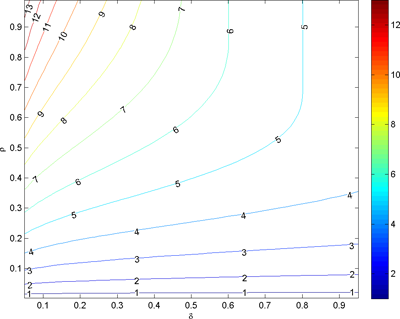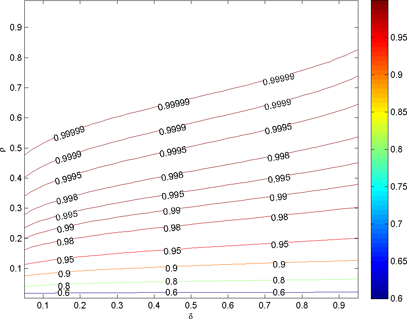Jared Tanner.

## Restricted Isometry Constants

Many of the compressed sensing recovery algorithms (decoders) are guaranteed to recover the sparsest solution to an underdetermined system of equations y = Ax provided the matrix A (encoder) acts sufficiently close to an isometry on sparse vectors. Let

χN(k) := {x ∈ RN : ‖xl0 ≤ k} ,

where ‖xl0 counts the number of nonzeros in x.

Let A be of size n × N with n < N. The Lower and Upper Restricted Isometry Constants (RICs) of A are defined as:

L(k, n, N; A) := minc ≥ 0 subject to (1 − c) ‖x22 ≤ ‖Ax22 for all x ∈ χN(k)

U(k, n, N; A) := minc ≥ 0 subject to (1 + c) ‖x22 ≥ ‖Ax22 for all x ∈ χN(k)

Gaussian matrices have the smallest known bound, with the tightest current bound provided in Improved bounds on Restricted Isometry Constants for Gaussian Matrices by Bubacarr Bah and Jared Tanner. Bounds on the Restricted Isometry Constants (RIC) for Gaussian matrices were first presented by Candes and Tao in Decoding by Linear Programming (IEEE Trans. Inform Theory, 51(12): 4203-4215). Their bounds were derived using a union bound and a concentration of measure bound on the Probability Density Function (PDF) of Gaussian/Wishart matrices. These bounds were improved upon by replacing the concentration of measure PDFs with the true PDFs of Gaussian/Wishart matrices. This make a substantial quantitative improvement, bringing the bounds to within a multiple of 1.87 of empirically observed RICs. These improved bounds are presented in Compressed Sensing: How sharp is the RIP?, by Jeffrey D. Blanchard, Coralia Cartis, and Jared Tanner. The more current tightest known bounds build upon the bounds of Blanchard, Cartis, and Tanner, achieving further improvements by exploiting dependencies in submatrices containing many of the same columns; these bounds are within a multiple of 1.57 of observed RICs.

Let ρn := k/n and δn := n/N be the compressed sensing over- and under-sampling ratios, respectively. Fix ε > 0, as n  ∞ with ρn  ρ ∈ (0,1) and δn  δ ∈ (0,1).

Prob(L(k, n, N; A) < L(δ, ρ) + ε)  1 and Prob(U(k, n, N; A) < U(δ, ρ) + ε)  1

Below are plots of the best known bounds, and functions which will calculate each of the above mentioned bound for your choice of ρ and δ. The different bounds are designated by the initials of the authors: BT for Bah and Tanner, CT for Candes and Tao, and BCT for Blanchard, Cartis, and Tanner. Note that smaller values are better, and that L is necessarily bounded by 1. The below forms are tested to give reliable answers over a range of δρ between zero and one. (Values close to zero or one may not be reliable.)

### Calculates U(δ,ρ) and L(δ,ρ)

Calculates U(δ,ρ) and L(δ,ρ)

### PlotsUpper bound on U(k,n,N;A) for A-Gaussian.Upper bound on L(k,n,N;A) for A-Gaussian.

Maintained by Jared Tanner. Last updated: Thursday, 19-Apr-2012 10:31:02 BST